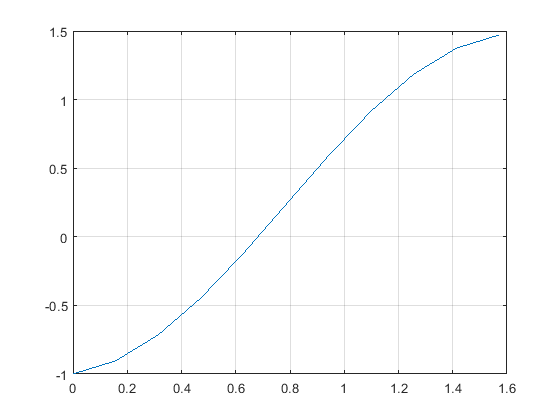# 2: Using fzero() to find the intersection of 2 functions

•• Carey Smith
• Oxnard College
$$\newcommand{\vecs}{\overset { \rightharpoonup} {\mathbf{#1}} }$$ $$\newcommand{\vecd}{\overset{-\!-\!\rightharpoonup}{\vphantom{a}\smash {#1}}}$$$$\newcommand{\id}{\mathrm{id}}$$ $$\newcommand{\Span}{\mathrm{span}}$$ $$\newcommand{\kernel}{\mathrm{null}\,}$$ $$\newcommand{\range}{\mathrm{range}\,}$$ $$\newcommand{\RealPart}{\mathrm{Re}}$$ $$\newcommand{\ImaginaryPart}{\mathrm{Im}}$$ $$\newcommand{\Argument}{\mathrm{Arg}}$$ $$\newcommand{\norm}{\| #1 \|}$$ $$\newcommand{\inner}{\langle #1, #2 \rangle}$$ $$\newcommand{\Span}{\mathrm{span}}$$ $$\newcommand{\id}{\mathrm{id}}$$ $$\newcommand{\Span}{\mathrm{span}}$$ $$\newcommand{\kernel}{\mathrm{null}\,}$$ $$\newcommand{\range}{\mathrm{range}\,}$$ $$\newcommand{\RealPart}{\mathrm{Re}}$$ $$\newcommand{\ImaginaryPart}{\mathrm{Im}}$$ $$\newcommand{\Argument}{\mathrm{Arg}}$$ $$\newcommand{\norm}{\| #1 \|}$$ $$\newcommand{\inner}{\langle #1, #2 \rangle}$$ $$\newcommand{\Span}{\mathrm{span}}$$$$\newcommand{\AA}{\unicode[.8,0]{x212B}}$$

## Using fzero() to find the intersection of 2 functions

Assume you have functions y1(x) and y2(x). Create a new function y3(x) = y1(x) - y2(x). The zero of y3(x) is the intersection of y1(x) and y2(x).

##### Example $$\PageIndex{4}$$ Find the intersection of cos(2x) and 3x

We will have 2 files. A test script file, and a function file.

% A. In the test script m-file, open a new figure and plot these 2 functions on it:
x = (0:0.05:1)*pi;
y1 = 0.3*x;
y2 = cos(2*x);
figure(1)
plot(x,y1)
hold on;
plot(x,y2)
grid on;
% We can see that one intersection of these functions is near x = 0.7 and y = 0.2%% Create a function file, f_3x_cos2x.m, that computes y = y1(x) - y2(x)

function y = f_3x_cos2x(x)
y1 = 0.3*x;
y2 = cos(2*x);
y = y1 - y2;
end

% The zero(s) of this single function is the intersection point(s) of the 2 functions.

% In the test script file, call fzero_3x_cos2x(x) and plot the result.

yy3 = fzero_3x_cos2x(x);
figure(2)
plot(x,yy3)
grid on;
title('y3 = y1 - y2')% We can see that one zero is near x = 0.7 and y3 = 0.0

%% Use Matlab's fzero function to find the roots of y3
x_solution = fzero(@f_3x_cos2x, 0.7) % near 0.7
% 0.6823

% Verify that this is a solution
y1b = 0.3*x_solution % 1st function
% 0.2047
y2b = cos(2*x_solution) % 2nd function
% 0.2047

%% Plot the solution point on the 1st figure
figure(1)
hold on;
plot(x_solution,y1b,'o')
plot(x_solution,y2b,'*').

##### Exercise $$\PageIndex{2}$$ fzero_exp

A. Set

x = (0 : 0.05 : 1)

B. Compute these 2 functions:

y1 = 0.7x

y2 = exp(-2*x)

C. Open a new figure and plot these 2 functions on it.

plot(x,y1)

hold on;

plot(x,y2)

The intersection of these 2 functions is the solution of this transcendental equation:

y1 = y2 or, equivalently, y1 - y2 = 0

D. Find the value of x near 0.5 that is a solution of this equation using the fzero() function as follows:

D1. Rewrite it in the form: fexp(x) = exp(-2*x) - 0.7*x, then create a function file called fexp.m that computes this.

D2. Solve it using the fzero() function, using @fexp as the function handle.